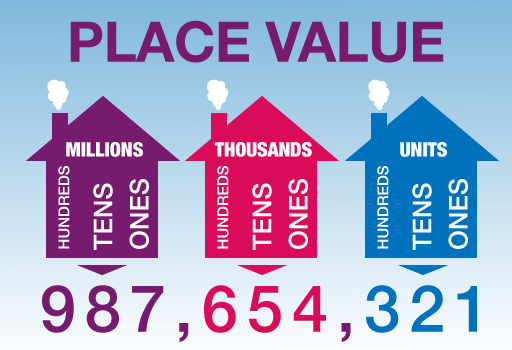Everyday maths 1

Start this free course now. Just create an account and sign in. Enrol and complete the course for a free statement of participation or digital badge if available.

Free course

# 1.1 Positive numbers and place valueFigure 1 Place value

Let’s look at positive numbers in more detail.

The place value of a digit in a number depends on its position or place in the number:

The value of 8 in 58 is 8 units.

The value of 3 in 34 is 3 tens.

The value of 4 in 435 is 4 hundreds.

The value of 6 in 6,758 is 6 thousands.

Look at the following example, which shows the place value of each digit in a seven-digit number.

## Example: What’s in a number?

Take the number 9,046,251. The value of each digit is as follows:

9 millions

0 hundred thousands

4 ten thousands (or 40 thousand)

6 thousands

2 hundreds

5 tens

1 unit

To make large numbers easier to read, we put them in groups of three digits starting from the right:

6532 is often written as 6,532 (or 6 532).

25897 is often written as 25,897 (or 25 897).

596124 is often written as 596,124 (or 596 124).

7538212 is often written as 7,538,212 (or 7 538 212).

## Activity 1: Working with place value

1. Write 4,025 in words.
Th H T U
4 0 2 5

4,025 in words is four thousand and twenty-five.

1. Write six thousand, four hundred and seventy-two in figures.
Th H T U
six four seven two

Six thousand, four hundred and seventy-two in figures is 6,472.

1. Here are the results of an election to be school governor at Hawthorn School:

Who won the election?

The person who wins the election is the person who gets the most votes.

To find the biggest number we need to compare the value of the first digit in each number. If this is the same for any of the numbers, then we need to go on to compare the value of the second digit in each number and so on.

• The value of the first digit in 436 is 4 hundreds.
• The value of the first digit in 723 is 7 hundreds.
• The value of the first digit in 156 is 1 hundred.
• The value of the first digit in 72 is 7 tens.

Comparing the values of the first digit in each number tells us that the biggest number is 723, so Sonia Cedar is the winner of the election.

FSM_1